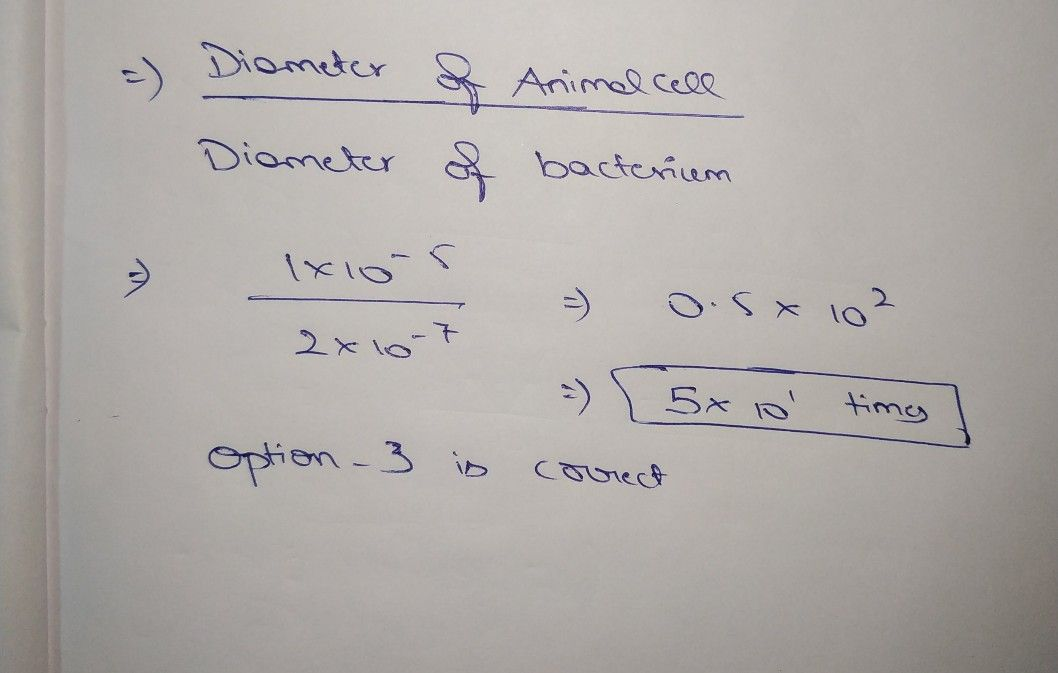Symbol
ProblemAn animal cell has a diameter of $1\times 10-5$ meter. $A$ bacterium has a diameter of $2\times 10-7$ meter. How many times as great is the diameter of the animal cell as the diameter of the bacterium? $O5\times 10^{3}$ times $O1\times 10^{2}$ times $O5\times 10^{1}$ times $O2\times 10^{2}$ times